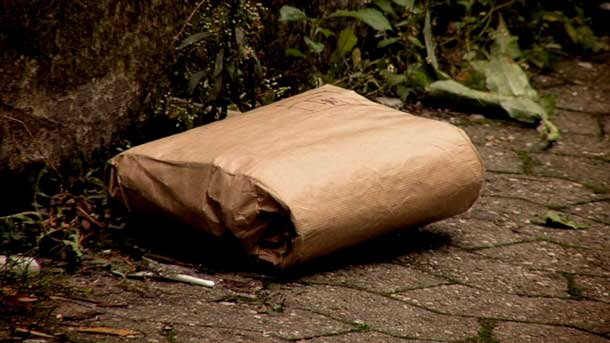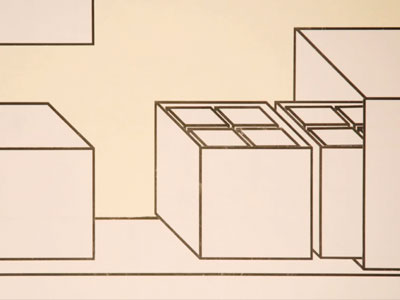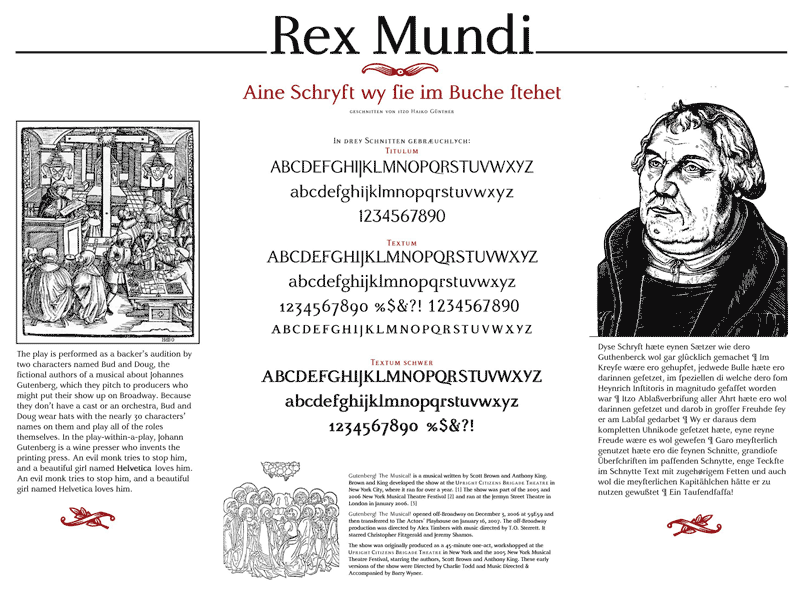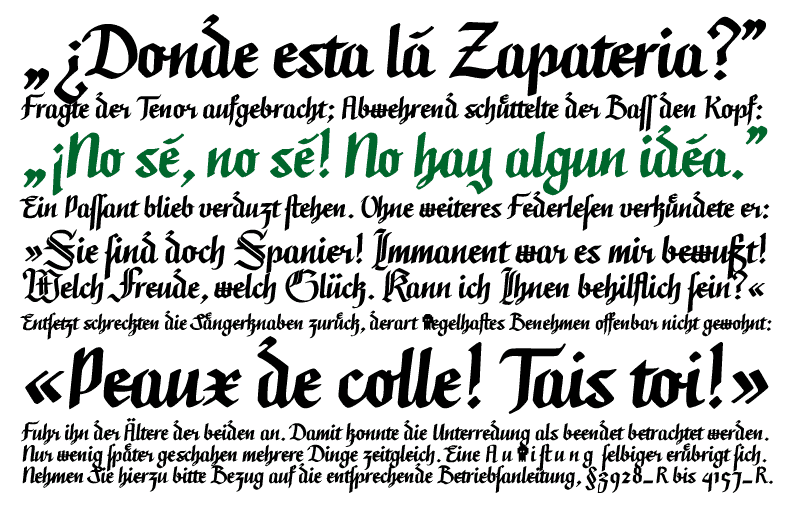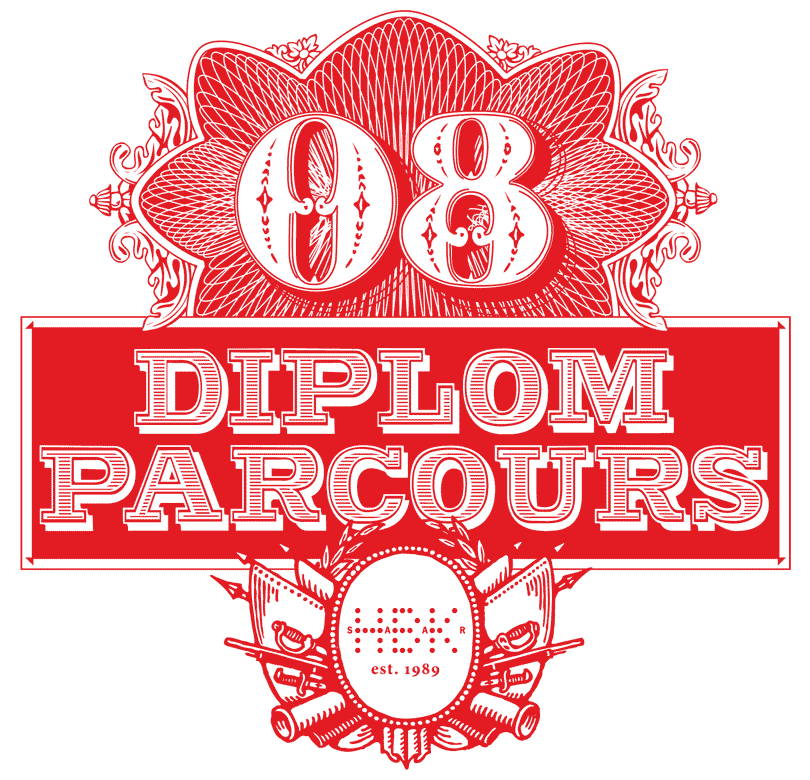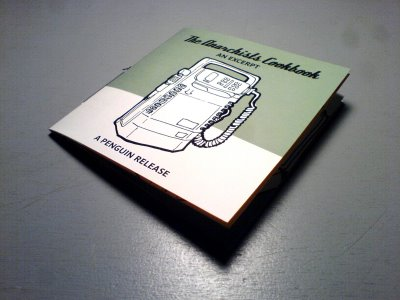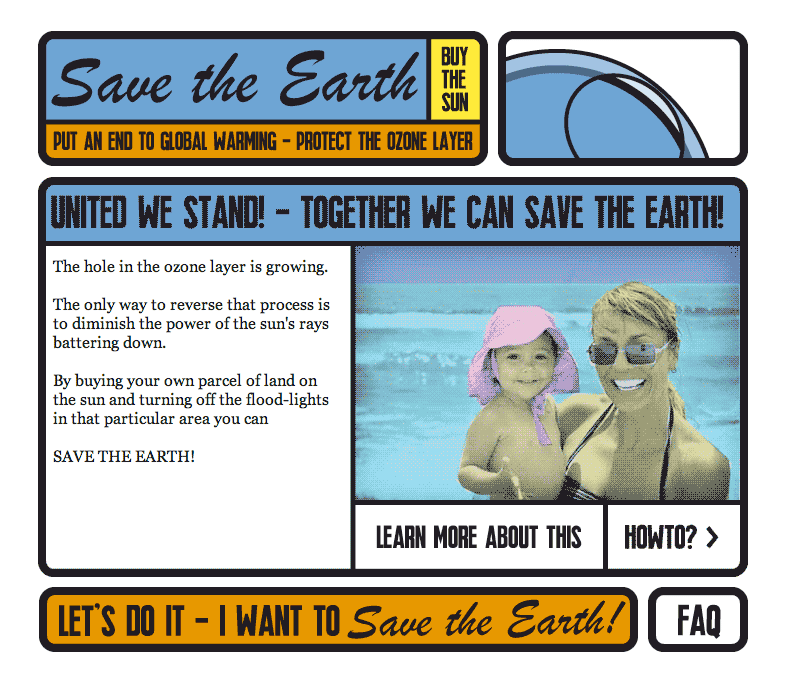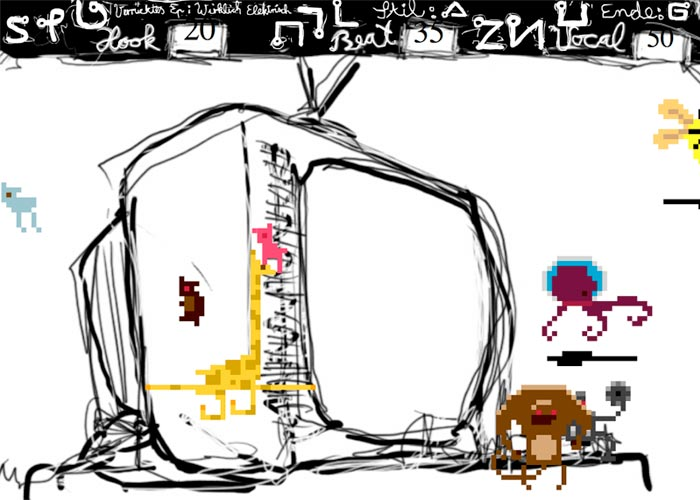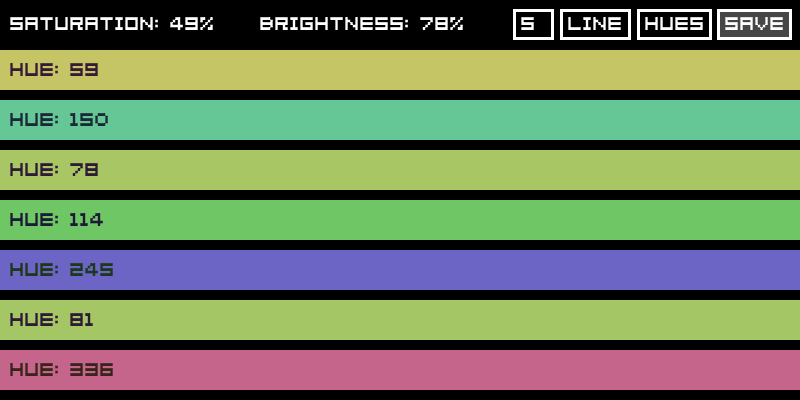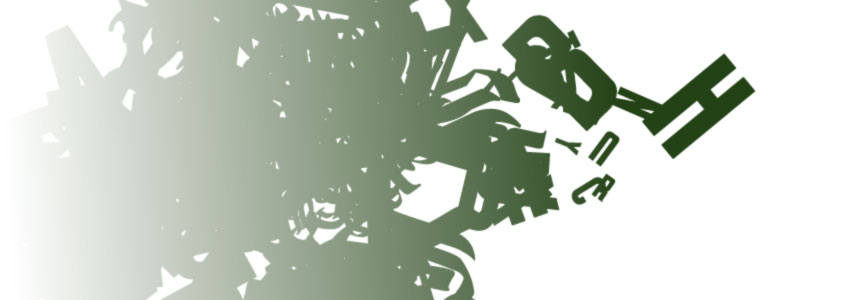x x x x x x x x x x x x x x x x x x x x x x x x x x x x x x x x x x x x x x x x x x x x x x x x x x x x x x x x x x x x x x x x x x x x x x x x x x x x x x x x x x x x x x x x x x x x x x x x x x x x x x x x x x x x x x x x x x x x x x x x x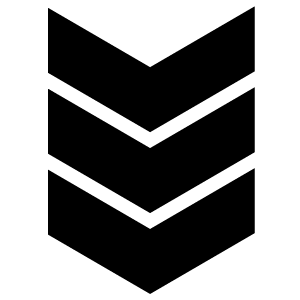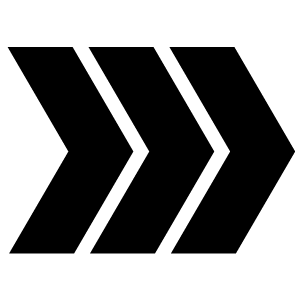››› main ››› the newest, the latest, the hippest, the hammer.
tha.fresh.shit.The ancient art of print-out soundeffects, revived.

category: ››› print ››› verunsicherungThis site is so fresh, I will have to leave it in the news section for ever.

category: ››› web ››› Kiosk Fonts is awesome!The nightmare of your sleepless nights, now in a graphic novel: THORVALD THE ALLMIGHTY!

category: ››› print ››› fear this pony!